## Optimizing Steering Car Paths with PyTorch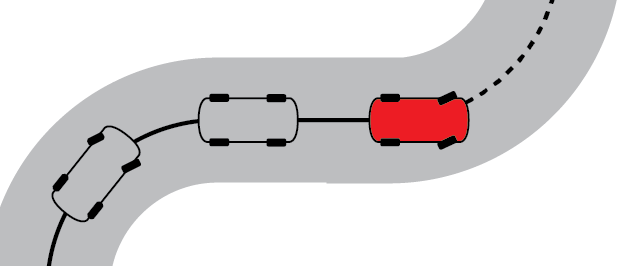Want to see another bizarre way to use PyTorch? If you've read some of my recent posts, you've seen the basic idea before. I'm using automatic differentiation and gradient descent, this time to optimize the path of a car. The model is similar to a Reeds-Shepp car, a simple steering car model. The state at time step $$i$$ is defined by a 2D position and an angle, $$(x_i, y_i, \theta_i)$$. In each step of the simulation, the car's state is updated based on an input consisting of speed and steering angle $$(v_i, \phi_i)$$.

$$x_{i+1} = x_i + v_i \cos{\theta_i} dt$$
$$y_{i+1} = y_i + v_i \sin{\theta_i} dt$$
$$\theta_{i+1} = \theta_i + v_i \phi_i dt$$

I simulated the car for $$n_\text{steps}$$ steps, with a target position and angle, $$(x_t, y_t, \theta_t)$$ and a cost function, $$c$$, which depended on the distance from the target position and the distance from the target angle in the final step.

$$c= \left(\theta_t - \theta_n\right)^2 +\left(x_t - x_n\right)^2 + \left(y_t - y_n\right)^2$$

I used automatic differentiation to find the derivates $$\frac{dc}{dv_i}$$ and $$\frac{dc}{d\phi_i}$$, which I used to update the inputs.

Check out some results. The animated plot below shows an example optimization, with the working solution evolving over time. The car starts at $$(0,0)$$, facing right and its target is $$(3,3)$$, facing down. The starting position and direction are represented by the red arrow and the target position and direction are represented by the green arrow.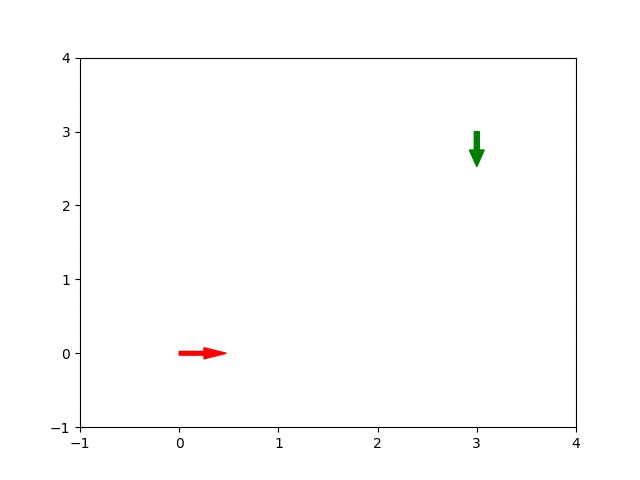In the first frame of the animation, the inputs are initialized to zero and the car does not move. In the ultimate solution, the car performs a wide left turn into its final position. The plots below shows the input that produced that trajectory and the evolution of the cost function.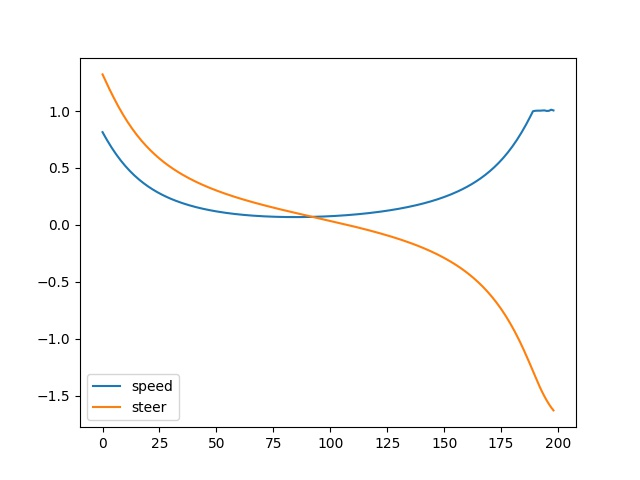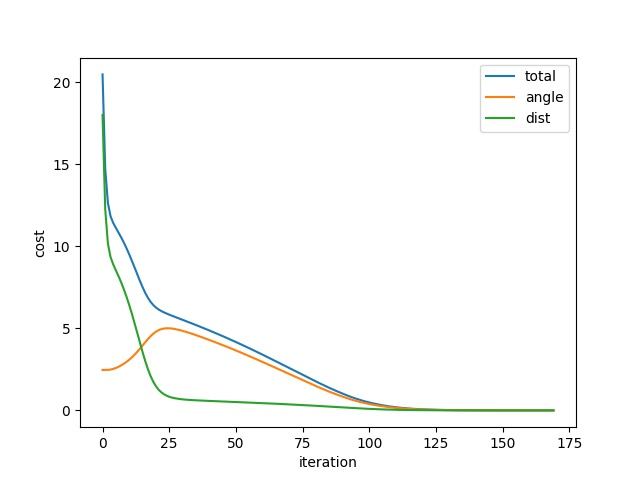Code below.

»

## Reinventing the Wheel: Discovering the Optimal Rolling Shape with PyTorch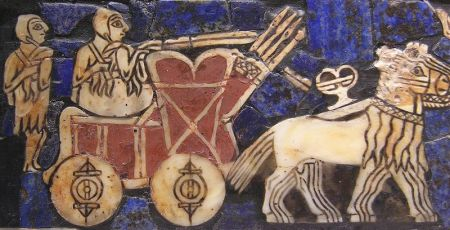It is thought that the wheel was invented more than five thousand years ago, yet modern machine learning tools were only developed in the past few decades. We have all wondered about this paradox at some point. How could ancient Sumerian wheel builders have calculated the ideal shape without modern computers and software? We may never know. Luckily, with today's technology, it is relatively easy to rediscover the wheel's optimal shape. I'll show you how I did it.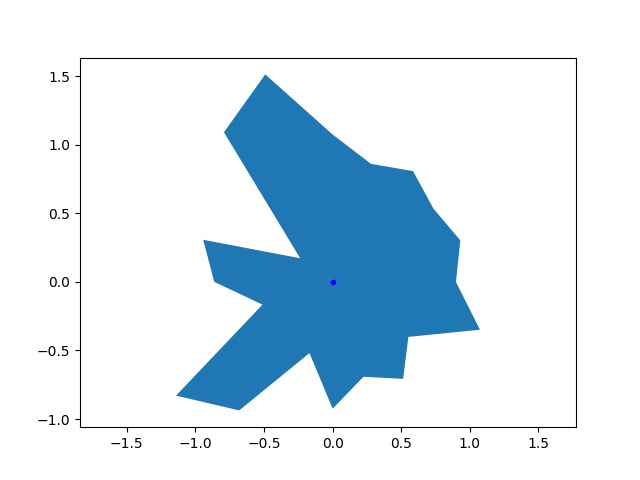The goal of this goofy project was to find the optimal shape of a wheel, which is probably a circle. I parameterized the wheel as a twenty sided polygon with a variable radius for each vertex, $$r_i$$. The performance of the wheel was based on the final speed achieved by an accelerating imaginary car, $$v_f$$. The wheel was driven with a constant torque, $$\tau$$, and no slipping. I used PyTorch to simulate the effect of a wheel and calculate the gradients in the final speed with respect to the radii, $$\frac{dv_f}{dr_i}$$. I used these gradients to update the radii using gradient descent. Above, you can see the shape of an initially randomly generated wheel the wheel evolving, along with the changing objecive function.

I represented the wheel with a relatively simple model. In each time step, I updated the speed of the car based on the force from the wheel, then rotated the wheel based on the speed. The lowest vertex was touching the ground and was fixed in space. The wheel generated a force on the car $$\tau/r_i$$, where $$r_i$$ is the radius of the vertex. A component of this force, shown in the diagram below, accelerated the car and increased its speed.Next, I think it would be fun to try surfaces that aren't flat. If I used that rolling square surface, would the optimal shape be a square?

»

## Haskell Integer to English

Inspired by a programming interview question I heard about, here's some cute code I wrote for converting (positive) integers to words. It breaks the number into groups of three digits, converts each group into English, and intersperses words like million and billion where necessary. I did the three digit number conversion using a cascading pattern matching system.

I had never thought about this problem much and was surprised by how many little quirks there are.

»

## Hanayama O'Gear Puzzle

Declan showed me a brain teaser his friend sent him. Apparently, it's known as the Hanayama O'Gear Puzzle. It consists of a bronze colored metal cube with a captive gear which can roll from face to face on the cube. The gear can also rotate between two directions on each face. Some edges of the cube are made so that the gear can't roll over them. One tooth of the gear and one face of the cube have a special cut-out. Only when the special tooth is engaged with the special face can the gear be freed. The object of the puzzle is to manipulate the gear into this orientation. Here's a video of the puzzle in action.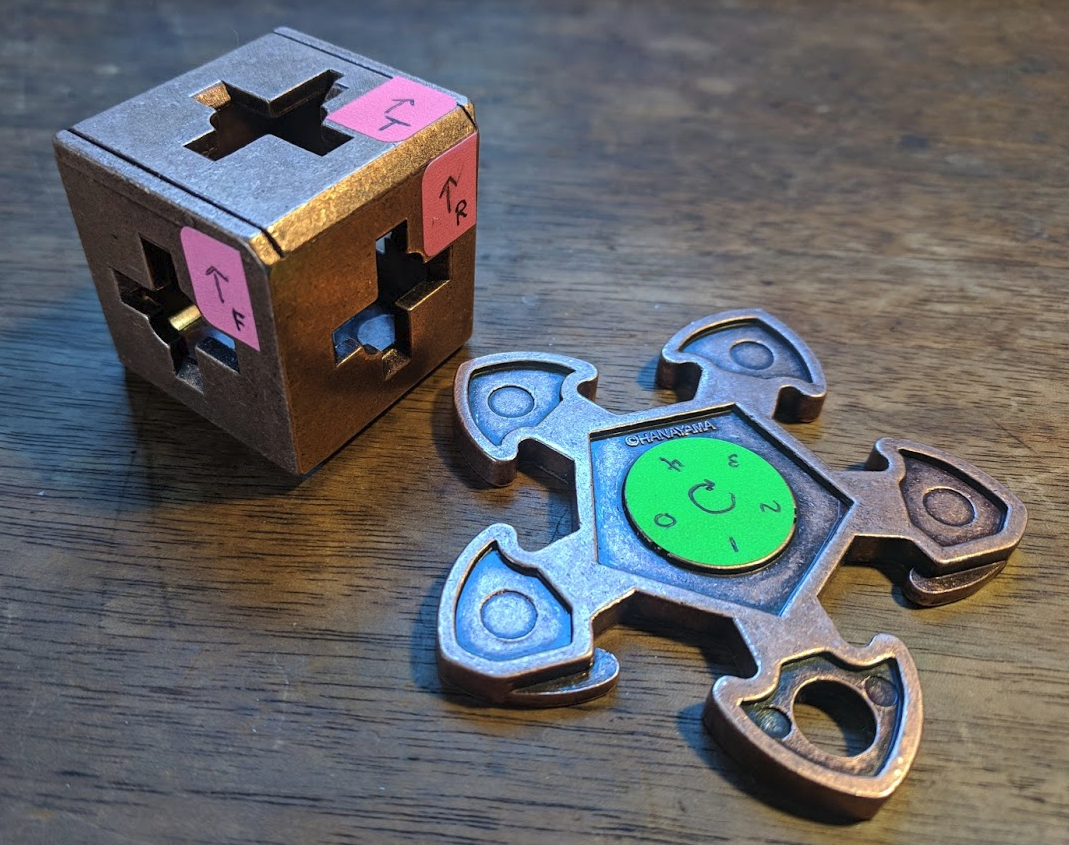I tried solving the puzzle for about fifteen minutes before deciding to use a computer. My basic strategy was to treat the problem as a graph search, where the nodes of the graph are the different states the cube and gear can be in. At any time, you can only do three things: rotate, roll forward, and roll backward. I figured that the limited set of moves and possible positions would prevent the search space from getting too big.

I marked up the cube with stickers, naming each face and defining its 'north' direction. I also marked the gear by labeling each tooth and setting a forward rotation direction.

I don't know Haskell very well, so I thought using it would be a fun challenge. Plus, I had Haskell guru Phil to ask for help and advice. A lot of the code is just defining the connectivity of the puzzle. I used a list to keep track of the links between different faces and a pattern-matched function to keep track of the possible rotations. I defined north on each face so that these rotations were always the same. A north-facing gear could only rotate to the east, and a south-facing one could only rotate west.

Beyond the trickiness of defining directions and connectivity, I just implemented a straightforward breadth-first search. The program creates a list of all states accessible from the starting state. Because the list is generated with successive expansions of the search frontier, it is naturally sorted by search depth. I take advantage of Haskell's lazy evaluation, creating an infinite list of accessible states but only ever calculating a few of them. Code below.

You can run it with a command like, solve (GearState Top South 4 []). This code, and a different Python solver I wrote are available in my Github.

»

## Couch Table

Matt and I needed a quick project for Christmas time. We came up with a small table to fit over the arm of a couch.It's an original design with a u-shaped base that allows the table to slide forward and out of the way of the arm rest by wrapping around the leg of the couch. We made it out of red oak with two small walnut accents. Plans below.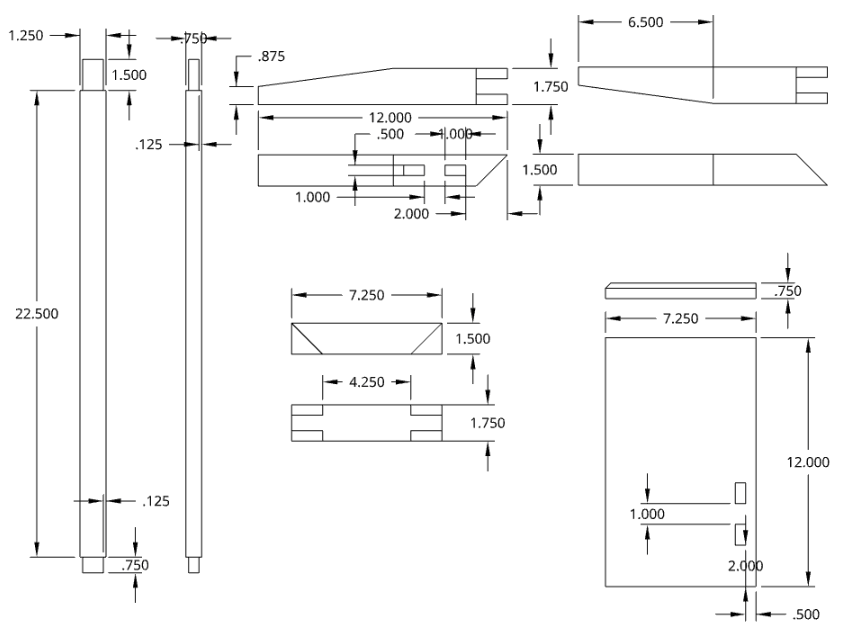First we built the base. We ripped 2" nominal thick red oak into 1.5" strips and chopped them to length. We cut shallow angles into the parallel parts of the base using a band saw and hand plane. We used hand tools to cut mitered bridle joint corners. These were the toughest part of the project, and we spent a lot of time fussing over the precise fit.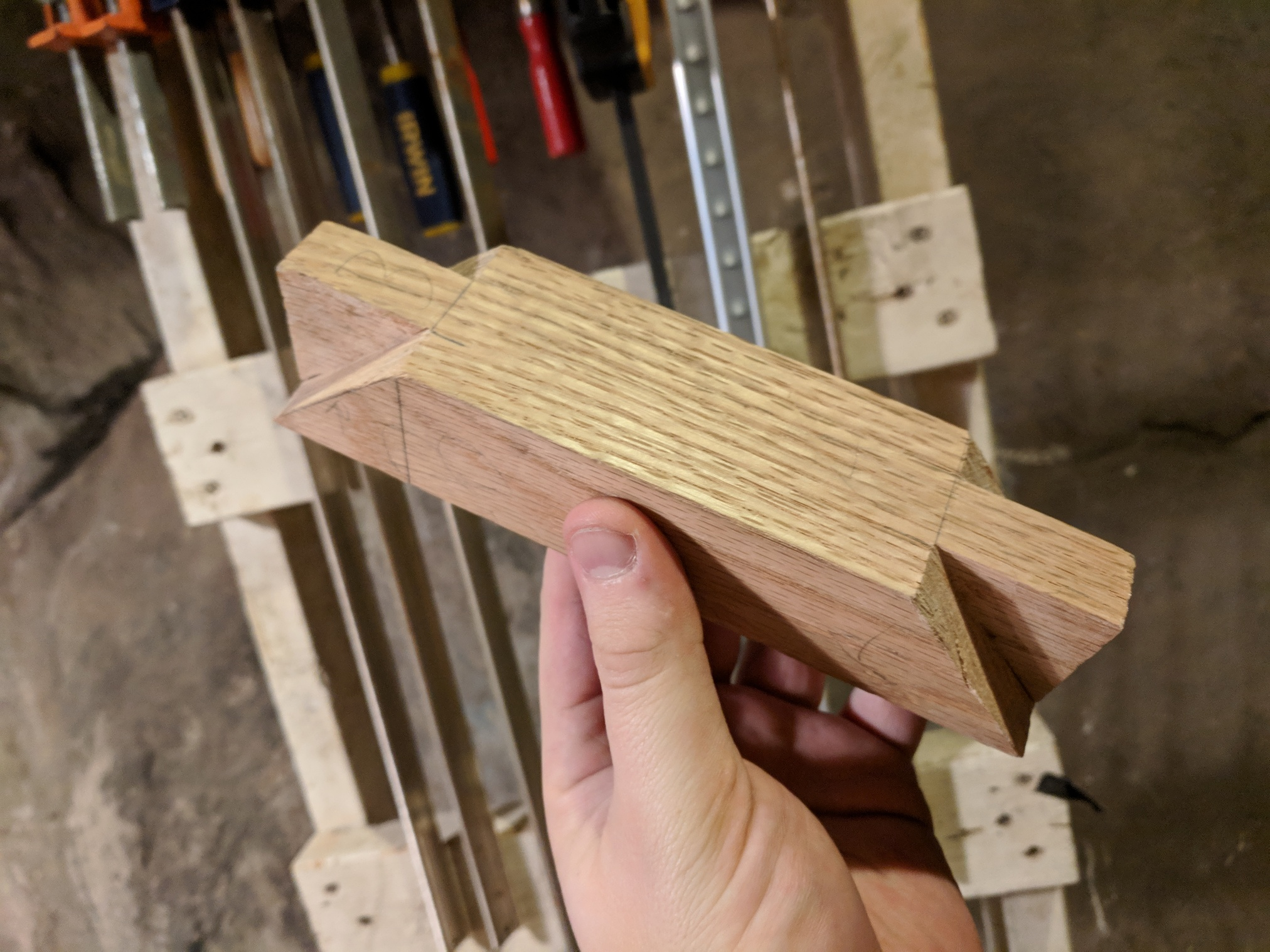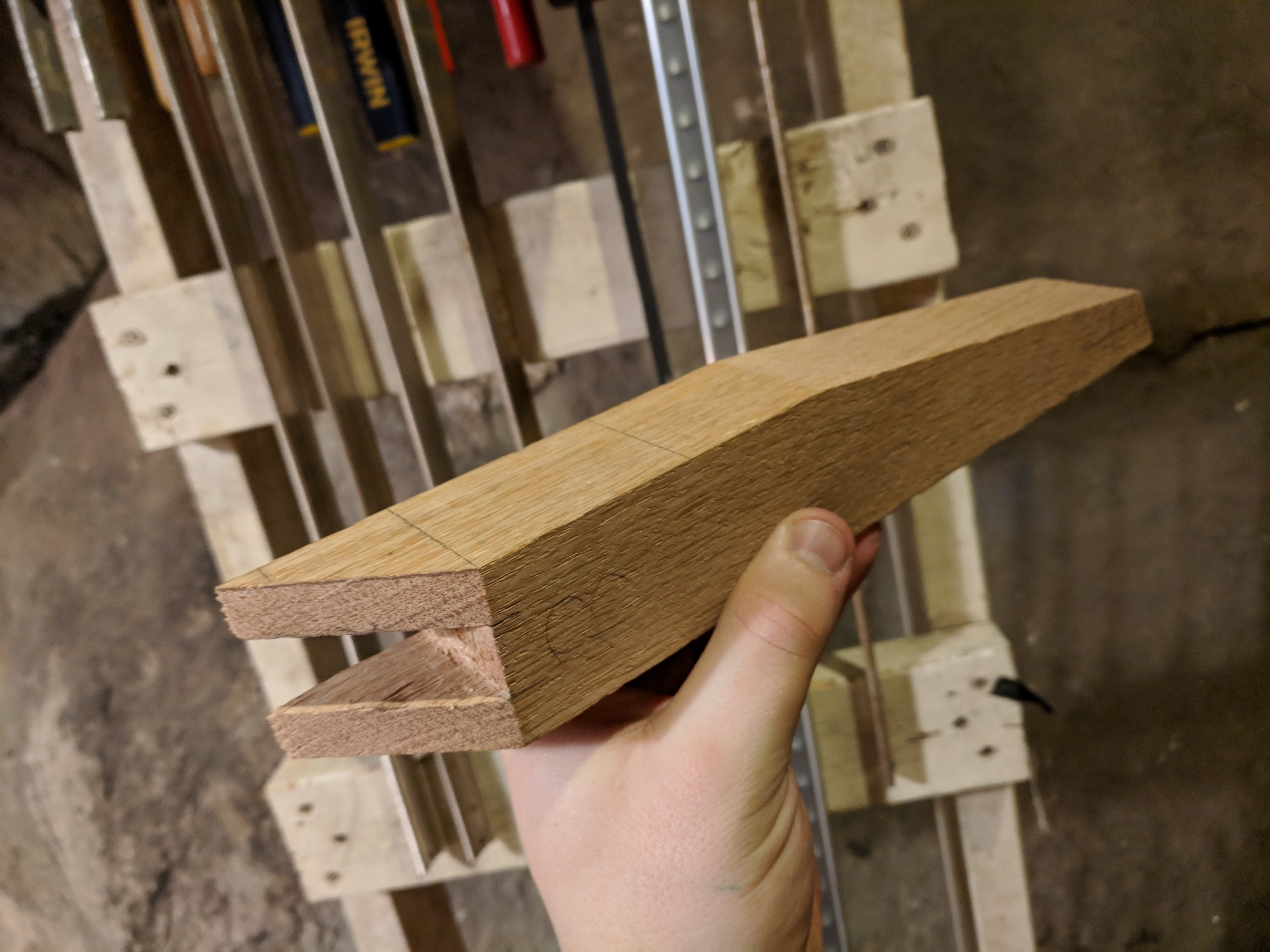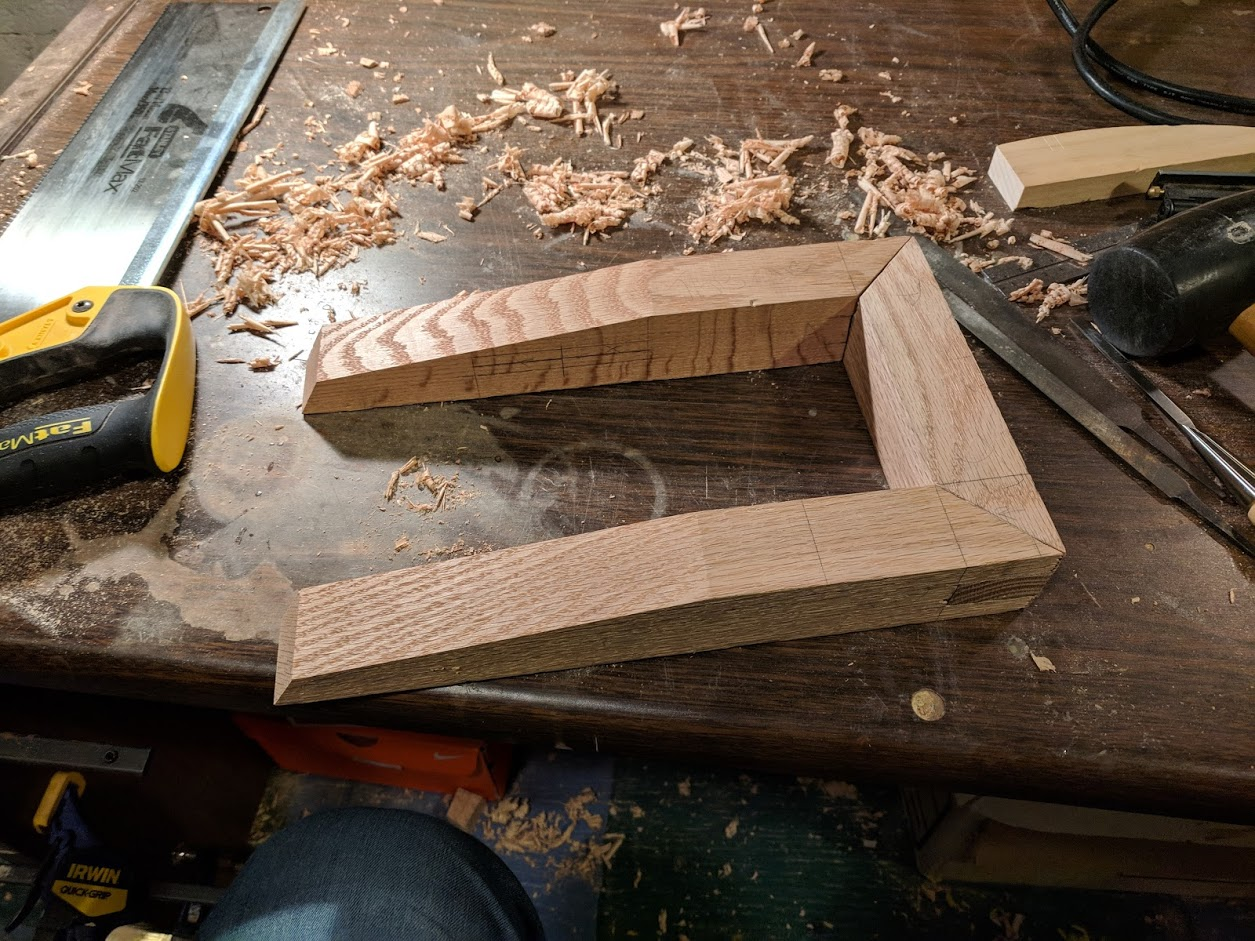We made the top of the table by chopping a piece of 1x12" red oak. We beveled three edges using a hand plane and cut the through mortices.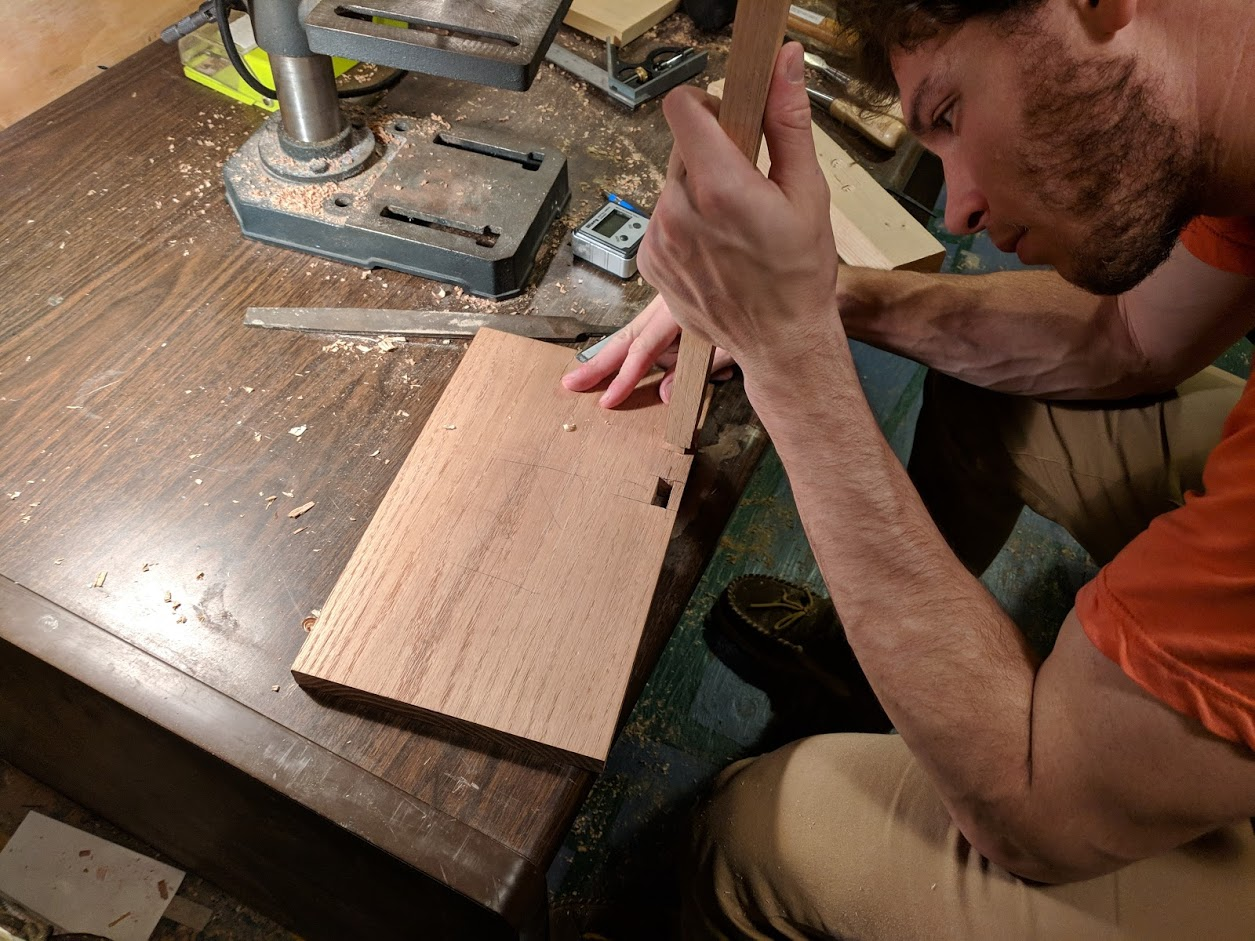Next, we made the vertical legs of the table. We ripped 1.25" strips of 1" nominal red oak and chopped them to length. We cut through tenons for the top and bottom with 1/8" shoulders.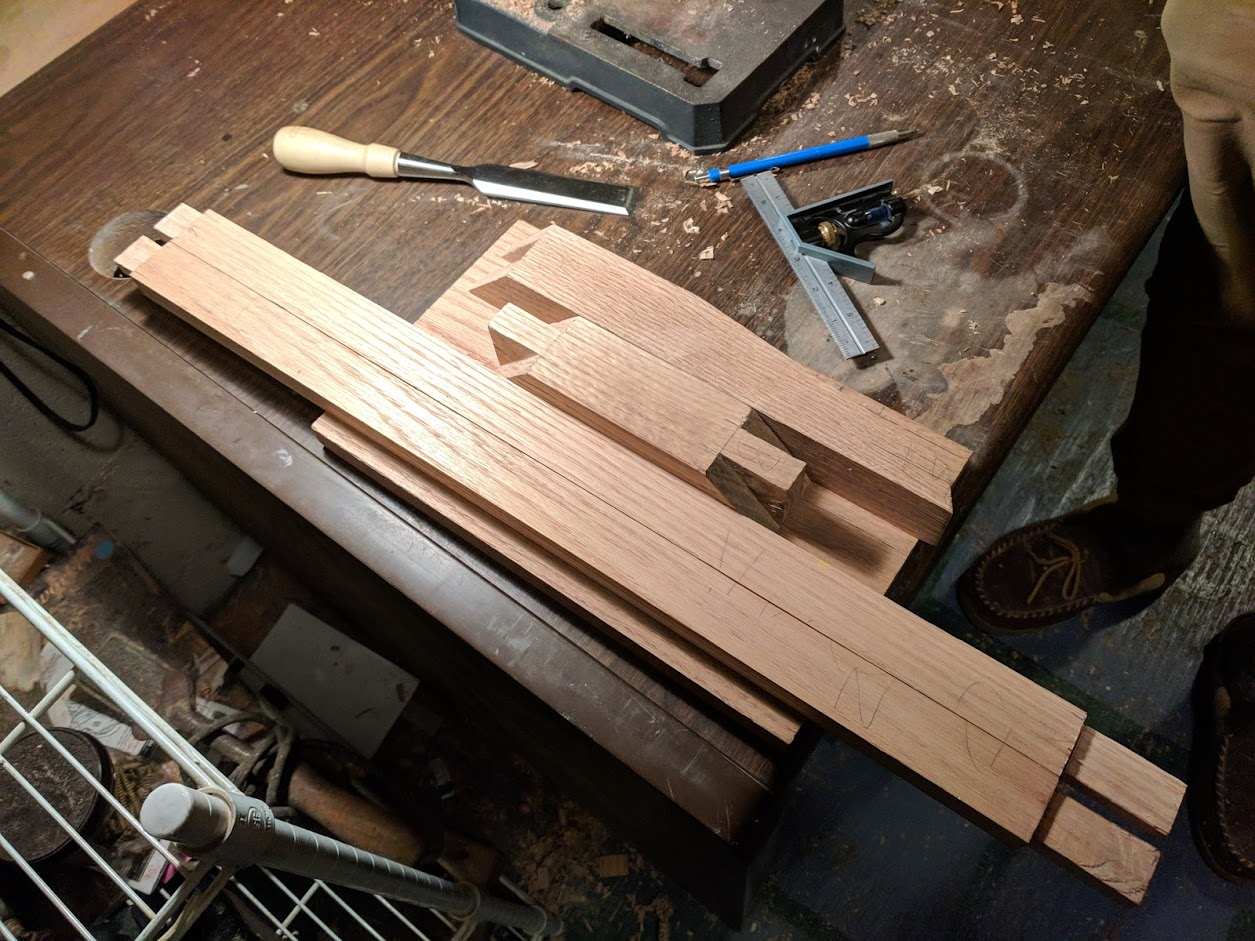We decided to try wedged tenons for a nice look on the top of the table. By eye, we cut some slim wedges out of walnut on the band saw. We also cut slots into the top tenon and flaired out the corresponding mortices slightly to accomodate the extra width from the wedges.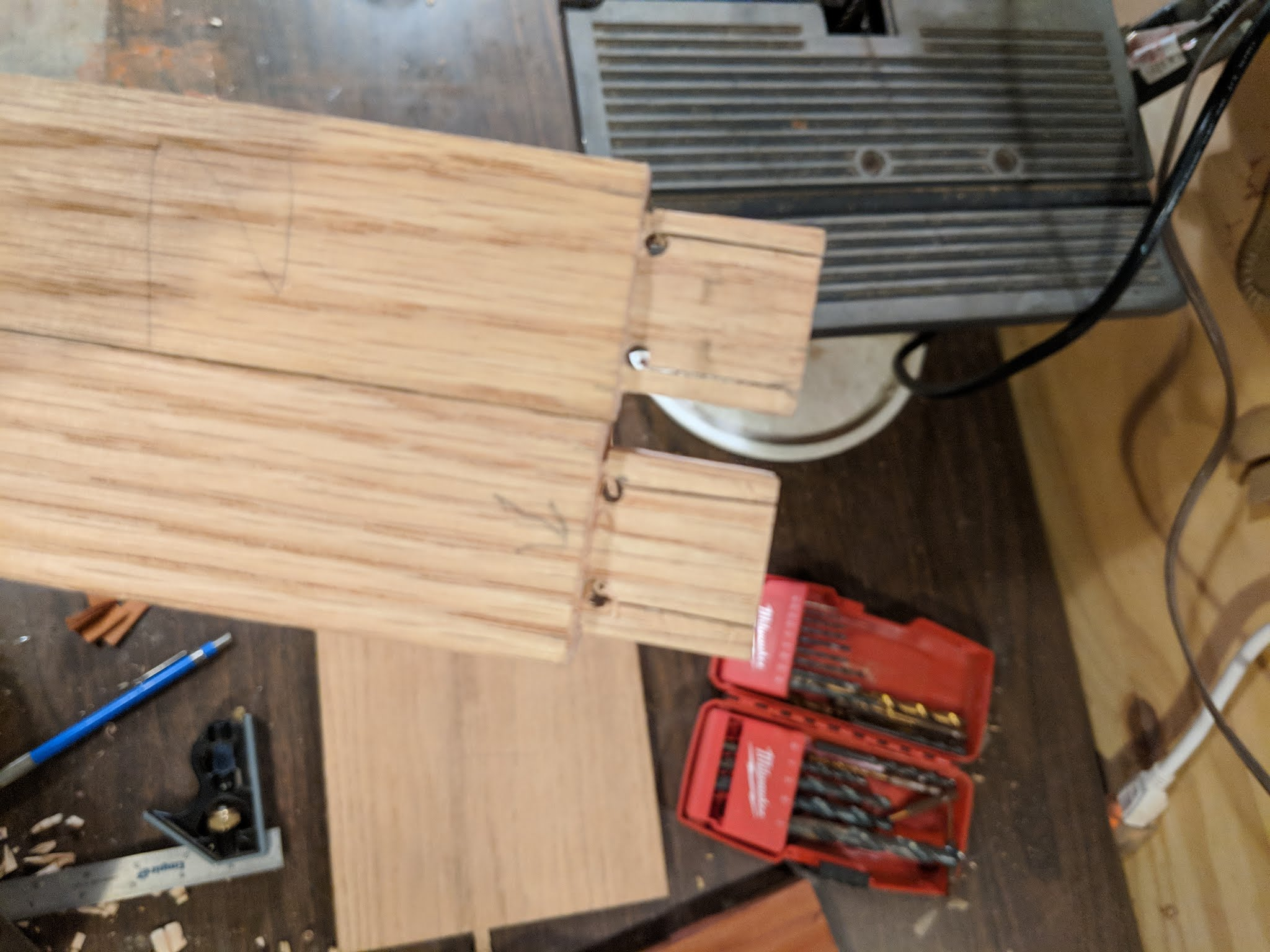We glued everything together and gently hammered the wedges in.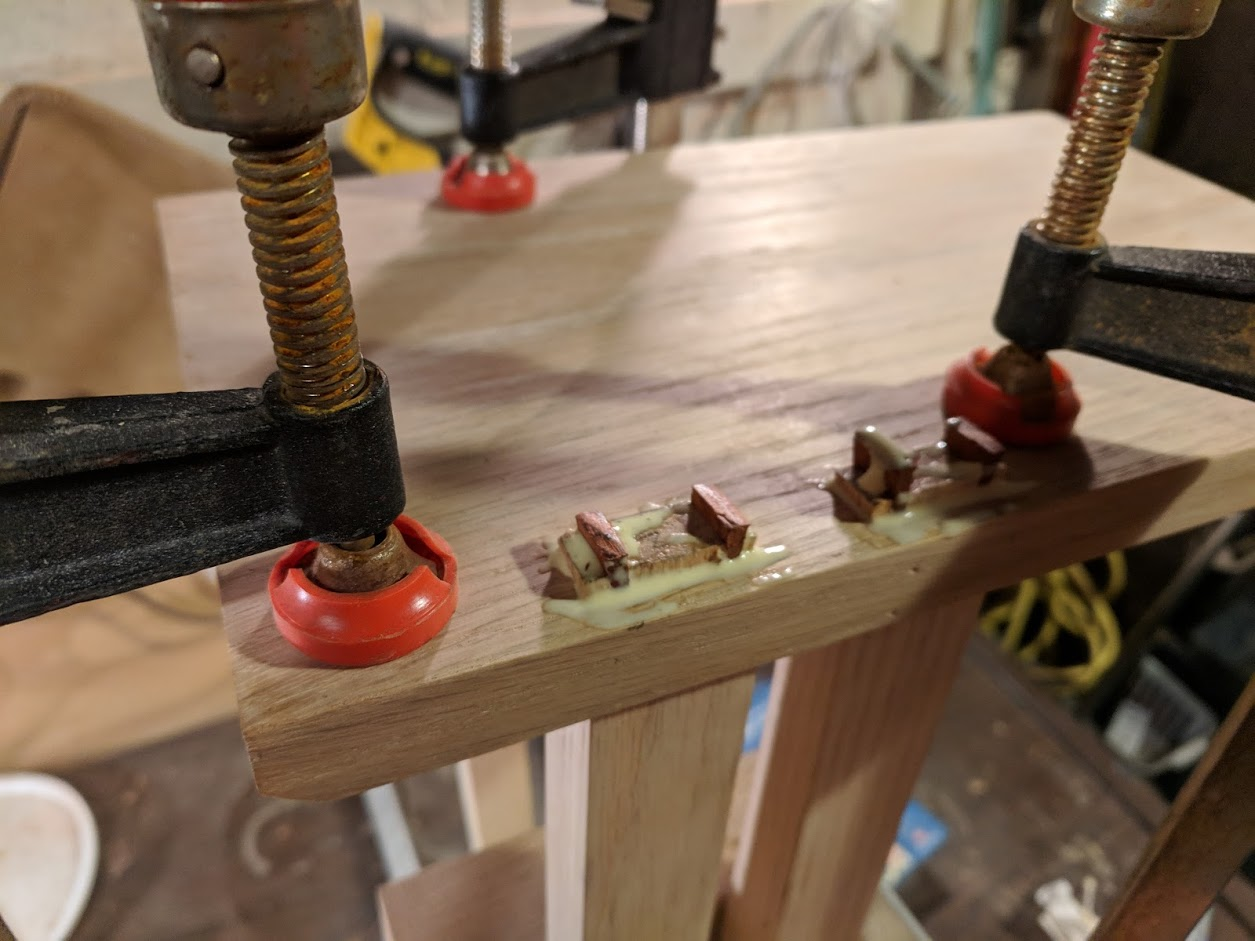Here's what they looked like after we sawed off the excess.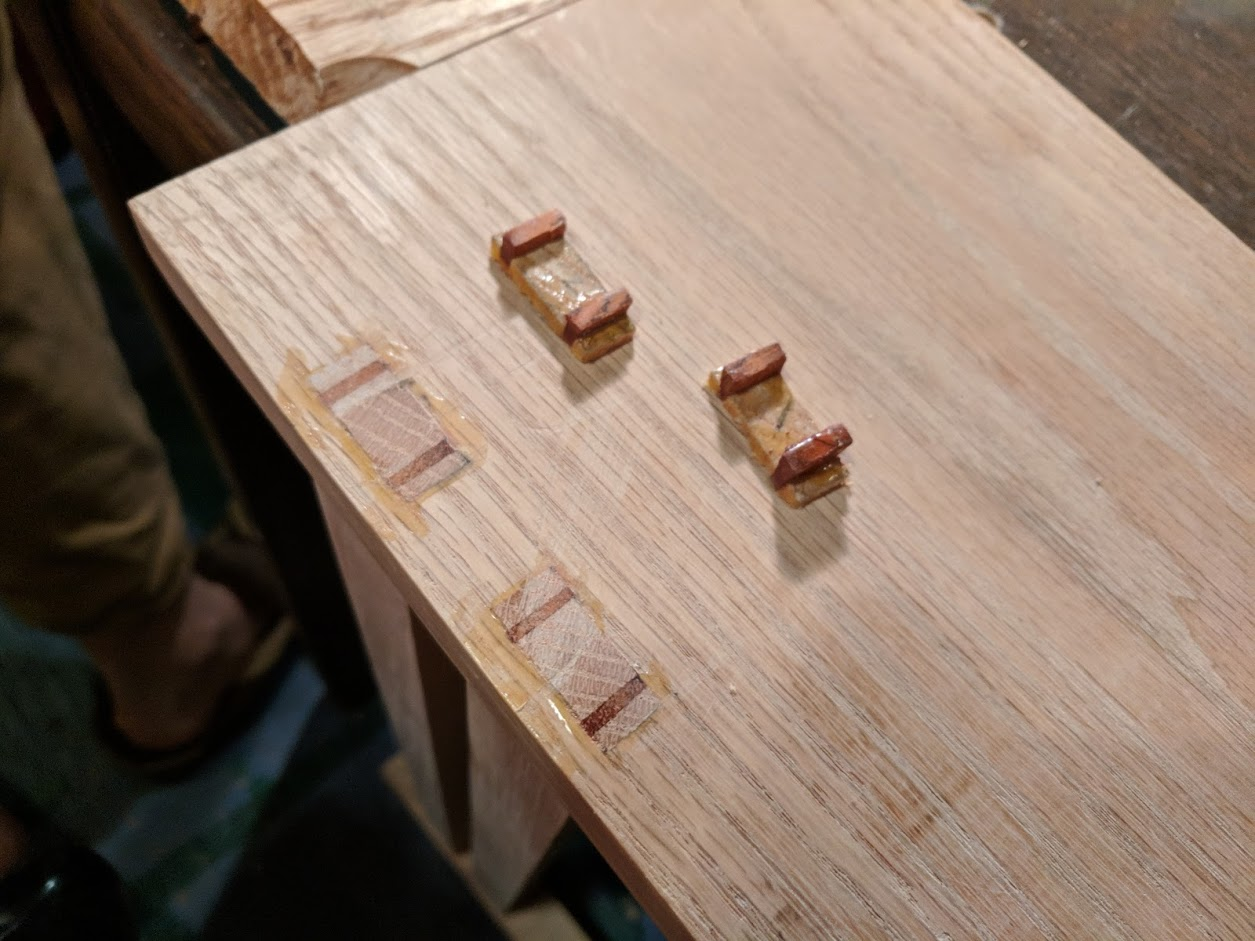Finally, we planed all of the joint surfaces flat and sanded down to 200 grit. For a finish, we decided to use danish oil. I highly recommend it. At least for red oak, it make the grain stand out and gives the whole thing a wonderful color. We also applied a layer of finishing wax.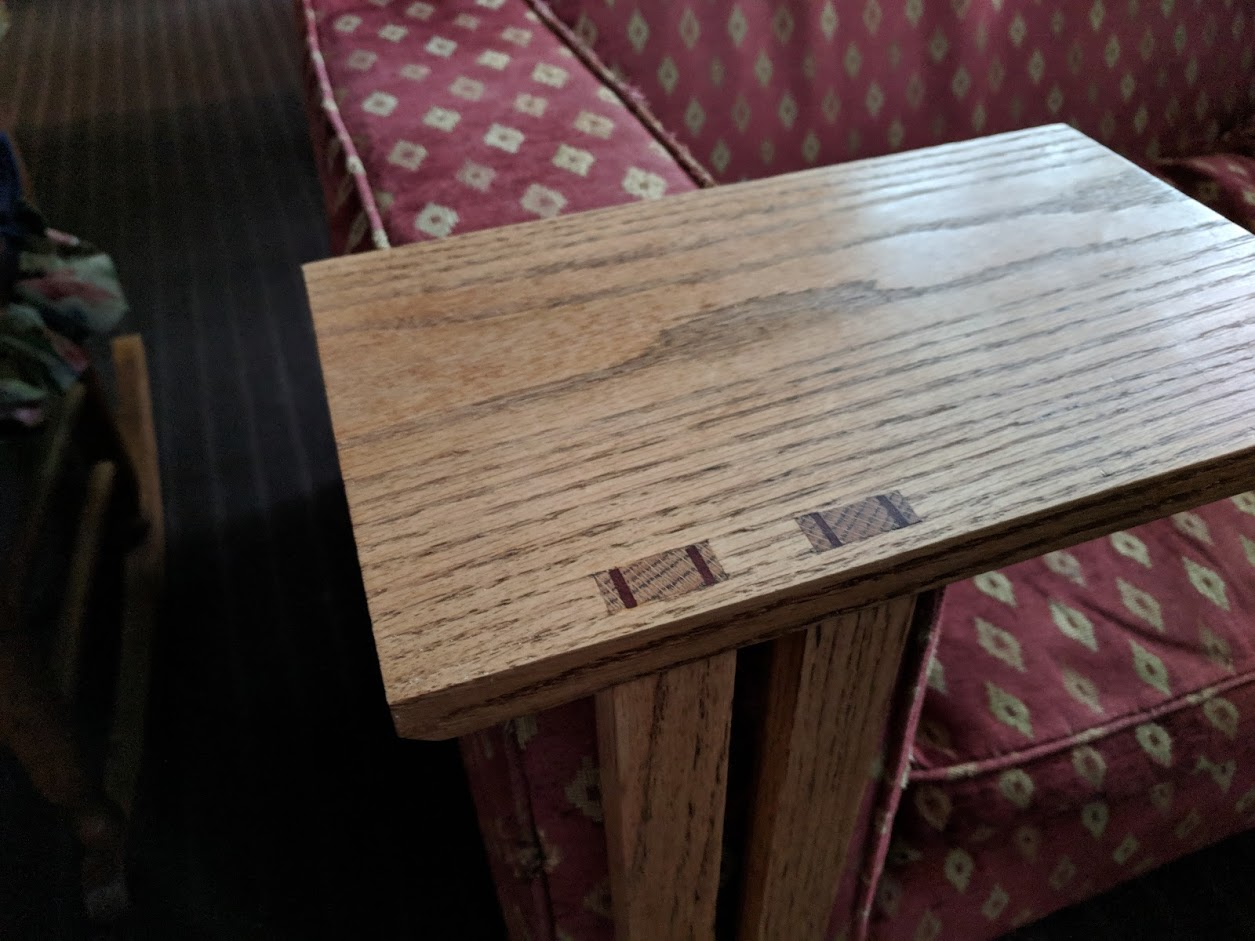This table was fun to build and came out looking good. It doesn't feel too unstable, either. With some simple length adjustments, it would be easy to fit this design to different couches and chairs.

»